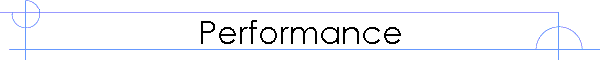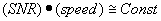Ultrasonics Applications and Solutions for Industry and Research

# Performance Envelope

### The Performance Envelope

The SIA-7 has almost nothing in common with any pulser receiver unit on the market today.  So how can we compare the performance of an SIA-7 to other ultrasonics equipment?  The only practical method is to define something called the performance envelope.  This is a boundary that defines whether an application is impossible, possible but difficult or practical.

The performance envelope can be ‘sketched’ using a simple formula.So what is the Signal to Noise Ratio (SNR) and how fast is the measurement process?  Can the measurements be improved to speed up an application?

### Signal to Noise Ratio

The only practical measure of the utility of an ultrasonic system (or any other sensor) is the effective signal to noise ratio.  The signal to noise ratio is a measure of the relative size of a signal to the noise background.  For any practical measurement the signal to noise ratio determines how useful an ultrasonic system will be.  The actual achieved signal to noise ratio can be influenced by many factors including how the system is set up, the choice of transducers and the types of signal processing used.

What does the signal to noise ratio describe?  In simple terms it is a measure of how large the signal is, relative to the background noise floor of the combined system.  The ratio is almost always expressed in dB so a value of 0.0dB corresponds to the case where the signal and noise levels are equal.  A signal to noise ratio of 0.0dB would mean that the signal would be difficult to detect and analyze because it is no larger than the surrounding noise.

A value of +20dB would indicate that the signal is 100 times larger than the noise floor.  Signal to noise ratios of +20dB or greater are considered to be ‘clean data’ that is easy to detect and analyze.

In engineering terms, there is always a trade-off between signal to noise ratio and the rate at which measurements can be made.  As a general guideline:More properly we should talk about the ‘acceptable SNR’.  For any given application there will be a minimum acceptable signal quality.  For a given ratio, there will be a maximum achievable speed.  This curve defines the envelope of the application and is useful to determine if a given application is practical for some particular purpose.

Home ] Up ]